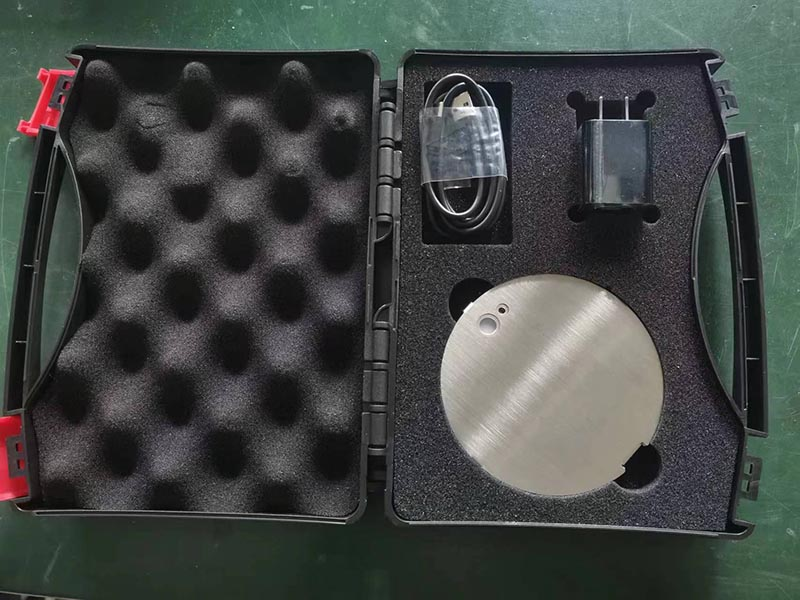# Measurement of Optical Power per Square Centimeter in Curing Equipment

Curing equipment is used to harden materials, such as resins, by exposing them to light. The optical power per square centimeter (mW/cm²) is a measure of the intensity of light in a given area. It is important to measure the optical power per square centimeter in curing equipment to ensure that the materials are cured properly.There are a number of ways to measure the optical power per square centimeter in curing equipment. One common method is to use a power meter. A power meter is an instrument that measures the amount of optical power in a given area. To measure the optical power per square centimeter, the power meter is placed in the path of the light beam and the reading is taken.

Another method for measuring optical power per square centimeter is to use a radiometer. A radiometer is an instrument that measures the radiant energy in a given area. To measure the optical power per square centimeter, the radiometer is placed in the path of the light beam and the reading is taken.

The choice of which method to use for measuring optical power per square centimeter depends on a number of factors, such as the type of curing equipment and the accuracy required.

Once the optical power per square centimeter has been measured, it can be used to calculate other quantities, such as the irradiance and the radiant exposure. Irradiance is the amount of optical power per unit area, and radiant exposure is the amount of optical power per unit area over a period of time.

The irradiance and radiant exposure are important quantities to consider when curing materials. The irradiance must be sufficient to cure the materials properly, but it must not be too high, as this could damage the materials. The radiant exposure must be sufficient to cure the materials in the desired amount of time, but it must not be too high, as this could also damage the materials.

The measurement of optical power per square centimeter in curing equipment is an important step in ensuring that materials are cured properly. By understanding the different methods for measuring optical power per square centimeter and the factors that affect the results, you can choose the best method for your application and get accurate measurements.

Here are some additional things to keep in mind about the measurement of optical power per square centimeter in curing equipment:

• The accuracy of the measurement depends on the type of instrument used and the conditions under which the measurement is made.
• The measurement should be made in a dark environment to avoid interference from ambient light.
• The distance between the light source and the detector should be kept constant.
• The beam profile of the light source should be uniform.